# OpenCV-Python 中的 K-Means 聚类

## 了解参数

### 输入参数

1. samples : 应该是 np.float32 数据类型，并且每个功能都应该放在一个列中。
2. nclusters(K) : 结束时所需的集群数量
3. criteria: 迭代终止标准。满足此条件时，算法迭代停止。实际上，这是一个有 3 个元素的 tuple。它们是( type, max_iter, epsilon ):
1. 终止标准的类型。 它有 3 个标志如下:
• cv.TERM_CRITERIA_EPS – 如果达到指定的精度 epsilon，则停止算法迭代。
• cv.TERM_CRITERIA_MAX_ITER – 在指定的迭代次数 max_iter 之后停止算法。
• cv.TERM_CRITERIA_EPS + cv.TERM_CRITERIA_MAX_ITER – 当满足上述任何条件时停止迭代。
1. max_iter – 一个指定最大迭代次数的整数。
2. epsilon – 要求的精度
4. attempts : 用于指定使用不同初始标记执行算法的次数的标志。算法返回产生最佳紧凑性的标签。该紧凑性作为输出返回。
5. flags : 该标志用于指定初始中心的采用方式。通常会使用两个标志: cv.KMEANS_PP_CENTERScv.KMEANS_RANDOM_CENTERS

### 输出参数

1. compactness : 每个点到其相应中心的平方距离之和。
2. labels : 标签数组（与前一篇文章中的’代码’相同），其中每个元素都标记为’0’，’1’…..
3. centers : 聚类中心数组

## 1. 只有一个特征的数据

import numpy as np
import cv2 as cv
from matplotlib import pyplot as plt

x = np.random.randint(25,100,25)
y = np.random.randint(175,255,25)
z = np.hstack((x,y))
z = z.reshape((50,1))
z = np.float32(z)
plt.hist(z,256,[0,256]),plt.show()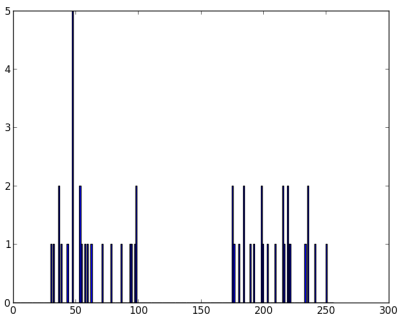# Define criteria = ( type, max_iter = 10 , epsilon = 1.0 )
criteria = (cv.TERM_CRITERIA_EPS + cv.TERM_CRITERIA_MAX_ITER, 10, 1.0)

# Set flags (Just to avoid line break in the code)
flags = cv.KMEANS_RANDOM_CENTERS

# Apply KMeans
compactness,labels,centers = cv.kmeans(z,2,None,criteria,10,flags)


A = z[labels==0]
B = z[labels==1]


# Now plot 'A' in red, 'B' in blue, 'centers' in yellow
plt.hist(A,256,[0,256],color = 'r')
plt.hist(B,256,[0,256],color = 'b')
plt.hist(centers,32,[0,256],color = 'y')
plt.show()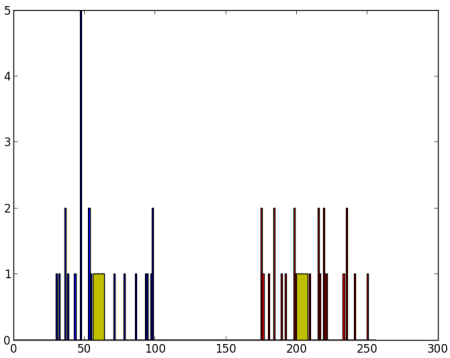## 2.具有多个特征的数据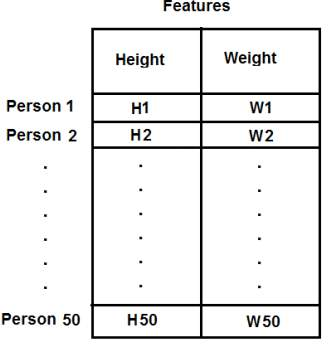import numpy as np
import cv2 as cv
from matplotlib import pyplot as plt

X = np.random.randint(25,50,(25,2))
Y = np.random.randint(60,85,(25,2))
Z = np.vstack((X,Y))

# convert to np.float32
Z = np.float32(Z)

# define criteria and apply kmeans()
criteria = (cv.TERM_CRITERIA_EPS + cv.TERM_CRITERIA_MAX_ITER, 10, 1.0)
ret,label,center=cv.kmeans(Z,2,None,criteria,10,cv.KMEANS_RANDOM_CENTERS)

# Now separate the data, Note the flatten()
A = Z[label.ravel()==0]
B = Z[label.ravel()==1]

# Plot the data
plt.scatter(A[:,0],A[:,1])
plt.scatter(B[:,0],B[:,1],c = 'r')
plt.scatter(center[:,0],center[:,1],s = 80,c = 'y', marker = 's')
plt.xlabel('Height'),plt.ylabel('Weight')
plt.show()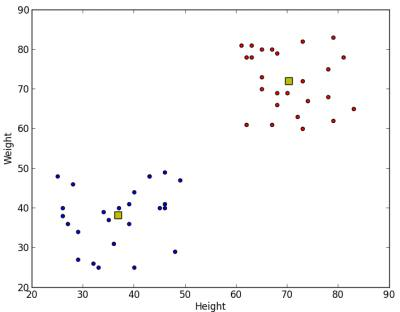## 3.色彩量化

import numpy as np
import cv2 as cv

Z = img.reshape((-1,3))

# convert to np.float32
Z = np.float32(Z)

# define criteria, number of clusters(K) and apply kmeans()
criteria = (cv.TERM_CRITERIA_EPS + cv.TERM_CRITERIA_MAX_ITER, 10, 1.0)
K = 8
ret,label,center=cv.kmeans(Z,K,None,criteria,10,cv.KMEANS_RANDOM_CENTERS)

# Now convert back into uint8, and make original image
center = np.uint8(center)
res = center[label.flatten()]
res2 = res.reshape((img.shape))

cv.imshow('res2',res2)
cv.waitKey(0)
cv.destroyAllWindows()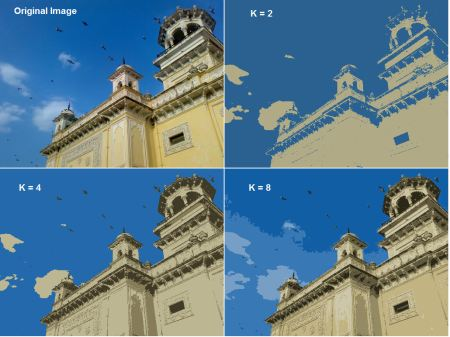• 微信订阅
• 回顶